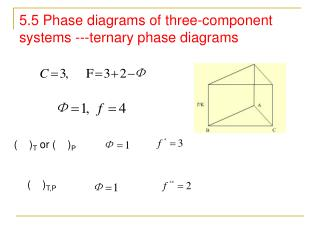DownloadDownload Presentation5.5 Phase diagrams of three-component systems ---ternary phase diagrams

# 5.5 Phase diagrams of three-component systems ---ternary phase diagrams

Télécharger la présentation## 5.5 Phase diagrams of three-component systems ---ternary phase diagrams

- - - - - - - - - - - - - - - - - - - - - - - - - - - E N D - - - - - - - - - - - - - - - - - - - - - - - - - - -
##### Presentation Transcript

1. 5.5 Phase diagrams of three-component systems ---ternary phase diagrams ( )T or ( )P ( )T,P

2. Equilateral triangle coordinates • The Gibbs Triangle

3. O, content point Mass fraction a＇ Content of A in the system b＇ Content of B in the system c＇ Content of A in the system

4. 22%B, 36%C, 42%A

5. 5.5.1 Composition characters for equilateral triangle coordinates d,e,f the mass fractions of A are equal Points on AD line, On line AD, D’ is closer to A than D, the mass fraction of A in D’ is more than that in D

6. D+E O Tie line The level rule for three-component systems O must be on the line DE

7. S b b S The mass fraction of A decrease, mA of A is separated from S (Φ=2, L+A) mA of A is added into the system

8. D + E + F H Centre-of-gravity rule of three-components system

9. 5.5.2 Partly miscible three-components liquid system O: isothermal consolute point aob: binoal curve a1-a4, b1-b4, a1-b1, a2-b2,a3-b3….. conjugate solution

10. B: H2O:Butanol:CH3COOH=30%:30%:40%=A+C A: 43%:22%:35% C: 14%:63%:23% A:C = 23:18

11. T - x1，x2 Single phase Two phase There are one couple of partly miscible components

12. Single phase Two-phase Two couples of partly miscible components Vinyl cyanide(A) water(B) ethanol(C)

13. Single phase L1 Two-phase Single phase L2

14. Single phase Two-phase There are three couples of partly miscible components Vinyl cyanide(A) water(B) ether(C)

15. Two-phase Φ=3 D,E, F Φ=1

16. Separation of liquid mixtures by extraction

17. Extraction Column

18. Φ=1 L Φ=2 B(s), L Φ=2 C(s), L Φ=3, B(s), C(s), F 5.5.3 Ternary water-salts systems Φ=3, the saturation solution of B and C

19. How to get pure B from mixtures of B and C Q-----R(L+B)

20. Effect of temperature on phase diagram T=298K T=373K

21. Purification of salt X(KNO3+NaNO3)…..D(298K)+KNO3…D(373K)…

22. X’: W……D‘……y……D

23. T-x

24. Φ=1, L Φ=2, L+Bi Φ=2, L+Pb Φ=2, L+Sn Pb-Sn-Bi trinary system Triple point Bi/Sn, Sn/Pb, Pb/Bi

25. Φ=3, L+Pb(S)+Sn(S) Φ=3, L+Bi(S)+Sn(S) Eutectic T decrease E4, L+Bi(S)+Sn(S)+Pb(S)

26. T decrease: A(Φ=1,L)… L+Bi(S) L+Bi(S)+Sn(S) L+Bi(S)+Sn(S)+Pb(S) Φ=4, f=0

27. The composition variation path

28. 5.6 The surfactant/oil/water trinary system

29. Homework • Y: P188 39 • Review the whole chapter